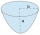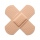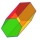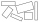# Circle arc

Circle segment has a circumference of 135.26 dm and 2096.58 dm2 area. Calculate the radius of the circle and size of central angle.

Result

r =  31.001 dm
f =  250 °

#### Solution:

$a \cdot \ r = 135.26 \ \\ r^2 \cdot \ a/2 = 2096.58 \ \\ \ \\ \ \\ r^2 \cdot \ (135.26/r) / 2 = 2096.58 \ \\ \ \\ 135.26r = 4193.16 \ \\ \ \\ r = 31.000739 \ \\ \ \\ a = 135.26/r = 135.26/31.0007 \doteq 4.3631 \ rad \ \\ \ \\ r = 31.0007 \doteq 31.0007 = 31.001 \ \text{ dm }$
$f = a \rightarrow \ ^\circ = a \cdot \ \dfrac{ 180 }{ \pi } \ \ ^\circ = 249.98846181458 \ \ ^\circ = 250 ^\circ = 249^\circ 59'18"$Our examples were largely sent or created by pupils and students themselves. Therefore, we would be pleased if you could send us any errors you found, spelling mistakes, or rephasing the example. Thank you!

Leave us a comment of this math problem and its solution (i.e. if it is still somewhat unclear...):Be the first to comment!Tips to related online calculators
Do you have a system of equations and looking for calculator system of linear equations?
Do you want to convert length units?

## Next similar math problems:

1. Eq triangle minus arcsIn an equilateral triangle with a 2cm side, the arcs of three circles are drawn from the centers at the vertices and radii 1cm. Calculate the content of the shaded part - a formation that makes up the difference between the triangle area and circular cuts
2. FlowerbedWe enlarge the circular flower bed, so its radius increased by 3 m. The substrate consumption per enlarged flower bed was (at the same layer height as before magnification) nine times greater than before. Determine the original flowerbed radius.
3. Circular railwayThe railway is to interconnect in a circular arc the points A, B, and C, whose distances are | AB | = 30 km, AC = 95 km, BC | = 70 km. How long will the track from A to C?
4. The parabolic segmentThe parabolic segment has a base a = 4 cm and a height v = 6 cm. Calculate the volume of the body that results from the rotation of this segment a) around its base b) around its axis.
5. Two patchesPeter taped the wound with two rectangular patches (one over the other to form the letter X). The area sealed with both patches at the same time had a content of 40cm2 and a circumference of 30cm. One of the patches was 8cm wide. What was the width of t
6. Rectangle fieldThe field has a shape of a rectangle having a length of 119 m and a width of 19 m. , How many meters have to shorten its length and increase its width to maintain its area and circumference increased by 24 m?
7. Uboid volumeCalculate the cuboid volume if the walls are 30cm², 35cm², 42cm²
8. Mysterious areaThe trapezoid ABCD is given. Calculate its area if the area of the DBC triangle is 27 cm2.
9. HexagonCalculate the surface of a regular hexagonal prism whose base edge a = 12cm and side edge b = 3 dm.
10. Diagonal intersectisosceles trapezoid ABCD with length bases | AB | = 6 cm, CD | = 4 cm is divided into 4 triangles by the diagonals intersecting at point S. How much of the area of the trapezoid are ABS and CDS triangles?
11. Two 2D shapesDecide which shapes have more area:   (a) a square of 8cm side; or   (b) two rectangles with sides 5cm and 15cm? Write result as 1 or 2 (rectangles)
12. Octagonal pyramidFind the volume of a regular octagonal pyramid with height v = 100 and the angle of the side edge with the plane of the base is α = 60°.
13. Tetrahedral pyramidDetermine the surface of a regular tetrahedral pyramid when its volume is V = 120 and the angle of the sidewall with the base plane is α = 42° 30´.
14. The schoolyardThe schoolyard had the shape of a square with an 11m side. The yard has been enlarged by 75 m2 and has a square shape again. How many meters was each side of the yard enlarged?
15. Paint cansHow many paint cans do we need to paint the floor in two rooms with dimensions of 6.8m x 4.5m and 6m x3.8m? One can arefor 6m².
16. RectanglesHow many different rectangles can be made from 60 square tiles of 1 m square? Find the dimensions of these rectangles.
17. Rectangular gardenThe perimeter of Peter's rectangular garden is 98 meters. The width of the garden is 60% shorter than its length. Find the dimensions of the rectangular garden in meters. Find the garden area in square meters.